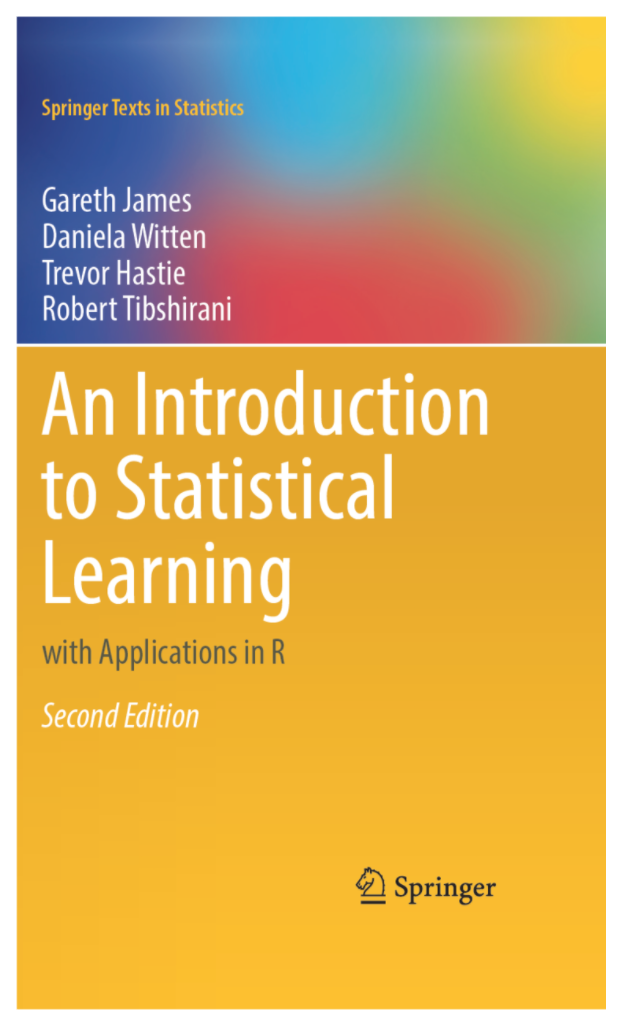## Session Objectives and Transferable Skills

• Provide a theoretical overview of Tree-based Machine Learning Techniques.
• Provide a technical and practical overview of Decision Trees for Classification and Regression Problems.
• Understand how to interpret these models and evaluate them.
• Understand how to design, develop, and interpret Tree-based Machine Learning Models in R.
• Using the packages tidyverse, caret & rpart.

## Schedule

• Introduction (5 minutes)
• Part 1: (45 minutes)
• Introduction to Machine Learning (Theory)
• What are tree-based models, what variations exist. (Theory)
• How to Prepare data for ML (Practical, Section 1)
• Growing your first decision Tree (Practical, Section 2)
• Break (5 minutes)
• Part 2: (35 minutes)
• How to read, and interpret, Decision Trees (Theory)
• Evaluating Trees in Practice (Practical, Section 3)
• Reviewing & Comparing models (Practical, Section 4)

## Introduction

• Content in this session will build upon content covered in this book.
• I won’t use any of the exercises or code examples covered in this book, so check them out for an alternative or further challenge!
• For Tree-Based Models, check out Chapter 8.## Introduction to Machine Learning

• Aim to address classification or regression problems

• Through predicting future outcomes based upon previous data.

• Techniques are classified either as:

• Supervised Techniques
• Unsupervised Techniques

## Introduction to Machine Learning

• Supervised Techniques:
• Tree-based Models
• Support Vector Machines (SVM)
• Neural Networks
• General Linear Models
• Generally problem focused approaches, with defined input & output variables

## Introduction to Machine Learning

• Unsupervised Techniques:
• K-Means or K-Medoids
• Gaussian Mixtures
• Neural Networks
• Generally exploratory focused approaches, with non-defined input & output variables

## What are Tree-Based Models

• Techniques which result in models which look generally like trees

• Of which decision trees, are the most simplistic form and can be considered the semantic base of all other models.

• Describe the outcome feature space, through grouping, segmentation or stratifying by the predictors provided,

• Achieved through generating binary splits in the feature space until the simplest spaces or groups are made